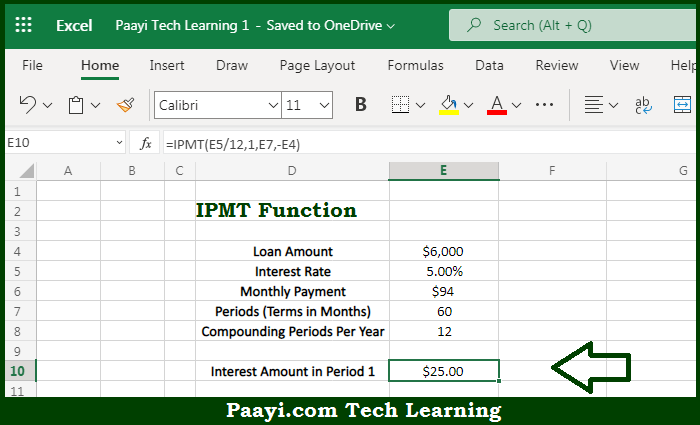# Learn How to Use Microsoft Excel IPMT Function

Written by | 0 Comments | 632 Views

In this article, you will learn how to use the Microsoft Excel IPMT function and its prime function in Microsoft Excel. You will also get to know the Microsoft Excel IPMT function return value and syntax with the help of some examples.

Microsoft Excel IPMT Function

The main purpose of the Microsoft Excel IPMT function is to get the interest for a given period. That implies, with the help of the IPMT function you can able to calculate the interest portion of given loan payment in a given payment period. For example, you can use IPMT to get the interest amount of payment for the first period, the last period, or any period in between. So, with the help of the IPMT function, you can able to get the interest for a given period.

Return Value of IPMT Function

The return value will be the interest amount.

Syntax of IPMT Function

=IPMT(rate, per, nper, pv, [fv], [type])

Where the arguments:

rate: This is the interest rate of the period.

per: This is the payment period of interest.

nper: This is the total number of payment periods.

pv: This is the present value, or the total value of all payments now.

fv: This is the cash balance desired after the last payment is made. Defaults to 0.

type: This is When payments are due. 0 = end of the period. 1 = beginning of the period. Default is 0 (optional).

## How to Use Microsoft Excel IPMT Function?So we know that Microsoft Excel IPMT function you can able to get the interest for a given period. That implies, with the help of the IPMT function you can able to calculate the interest portion of given loan payment in a given payment period. For example, you can use IPMT to get the interest amount of payment for the first period, the last period, or any period in between. So, with the help of the IPMT function, you can able to get the interest for a given period.